# CHEMICAL REACTIONS DESCRIBING CHEMICAL CHANGE n In a

• Slides: 17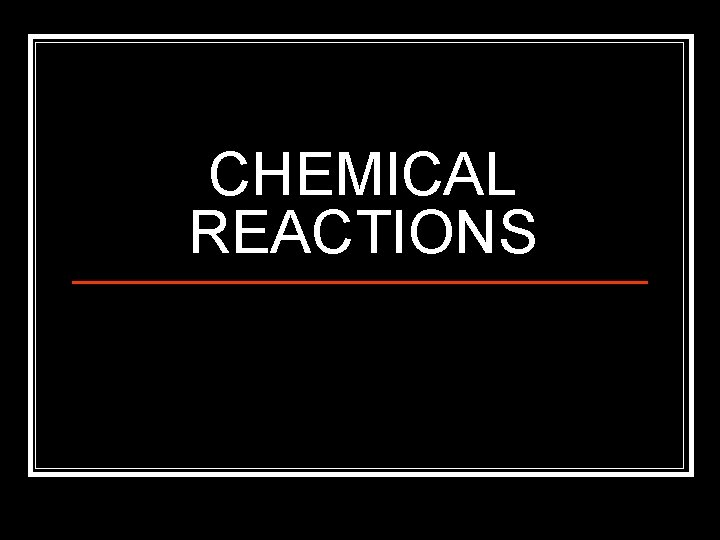CHEMICAL REACTIONS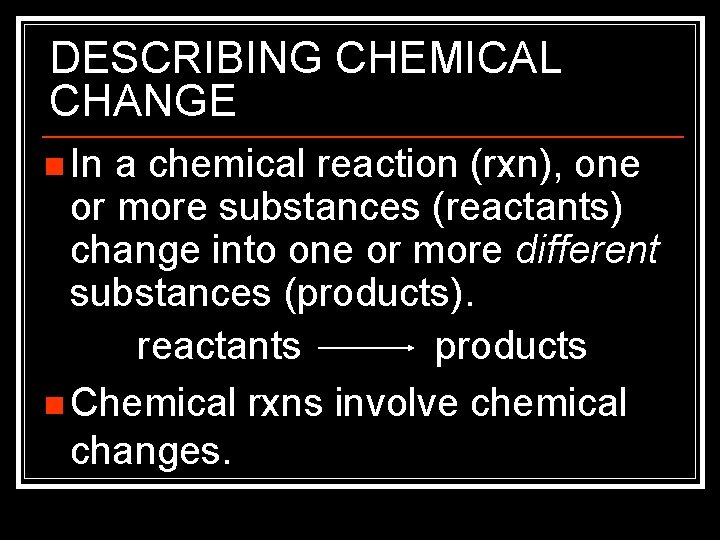DESCRIBING CHEMICAL CHANGE n In a chemical reaction (rxn), one or more substances (reactants) change into one or more different substances (products). reactants products n Chemical rxns involve chemical changes.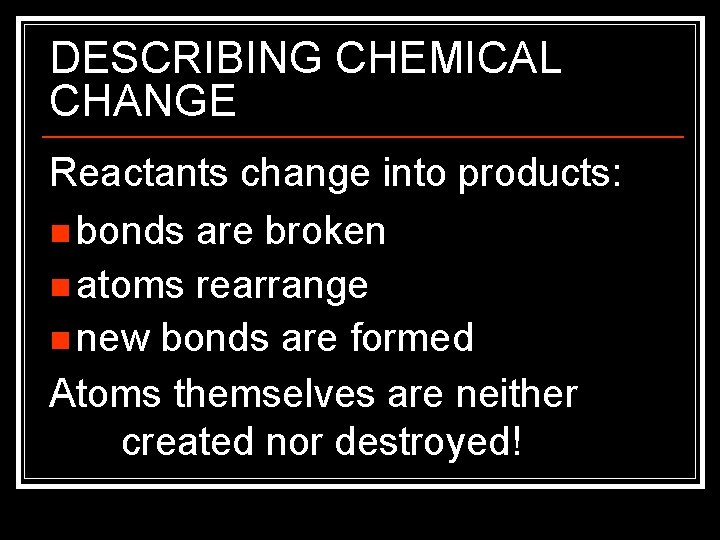DESCRIBING CHEMICAL CHANGE Reactants change into products: n bonds are broken n atoms rearrange n new bonds are formed Atoms themselves are neither created nor destroyed!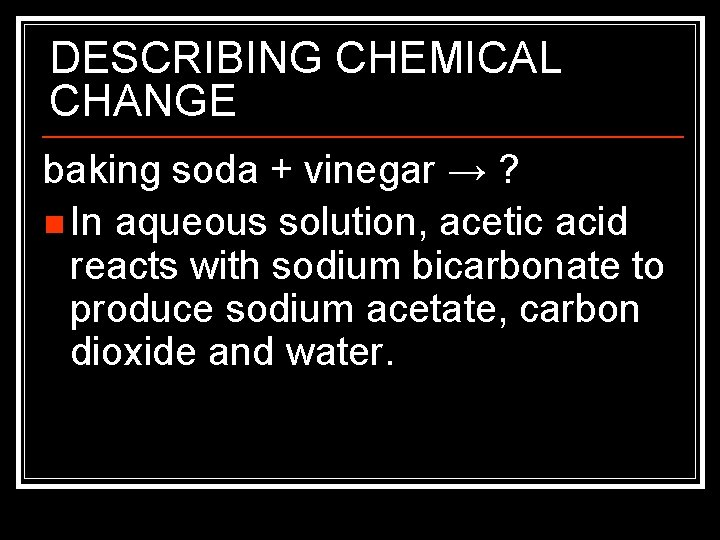DESCRIBING CHEMICAL CHANGE baking soda + vinegar → ? n In aqueous solution, acetic acid reacts with sodium bicarbonate to produce sodium acetate, carbon dioxide and water.DESCRIBING CHEMICAL CHANGE sodium bicarbonate + aqueous acetic acid sodium acetate + carbon dioxide + water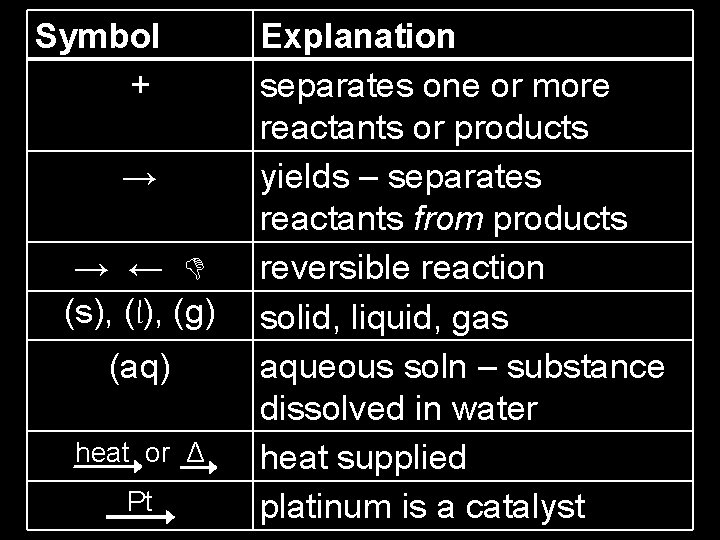Symbol + Explanation separates one or more reactants or products → yields – separates reactants from products → ← reversible reaction (s), (l), (g) solid, liquid, gas (aq) → aqueous soln – substance → dissolved in water heat or Δ heat supplied Pt platinum is a catalyst heat PtCHEMICAL EQUATIONS physical states of the substances indicated by symbol in parentheses after the formula C 12 H 24(s) + O 2(g) → CO 2(g) + H 2 O (g)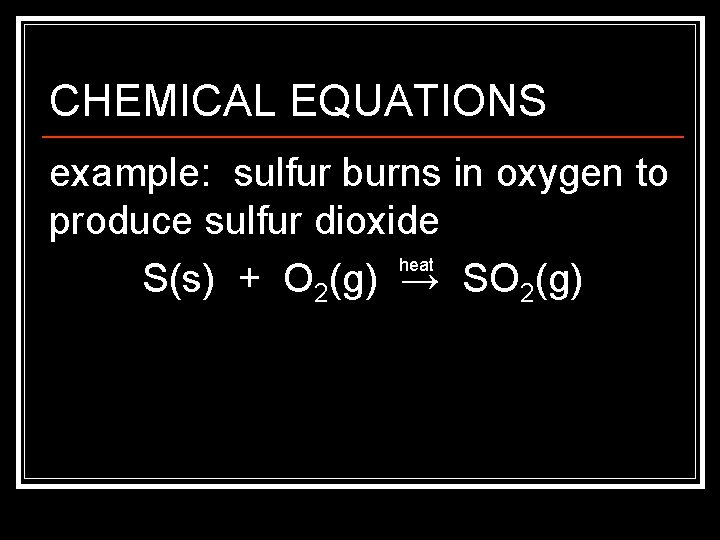CHEMICAL EQUATIONS example: sulfur burns in oxygen to produce sulfur dioxide heat S(s) + O 2(g) → SO 2(g)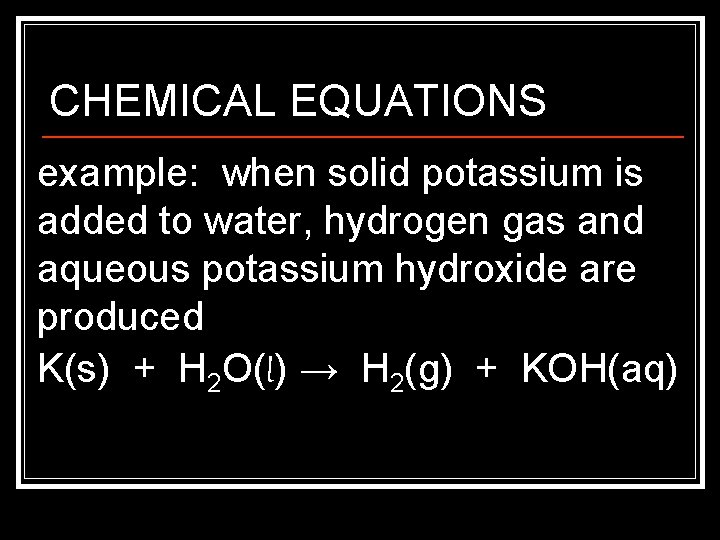CHEMICAL EQUATIONS example: when solid potassium is added to water, hydrogen gas and aqueous potassium hydroxide are produced K(s) + H 2 O(l) → H 2(g) + KOH(aq)BALANCING CHEMICAL EQUATIONS burning candle equation: C 12 H 24(s) + O 2(g) → CO 2(g) + H 2 O(l) when one molecule of wax reacts, does one molecule of CO 2 form? C 12 H 24(s) + 18 O 2(g) → 12 CO 2(g) + 12 H 2 O(l)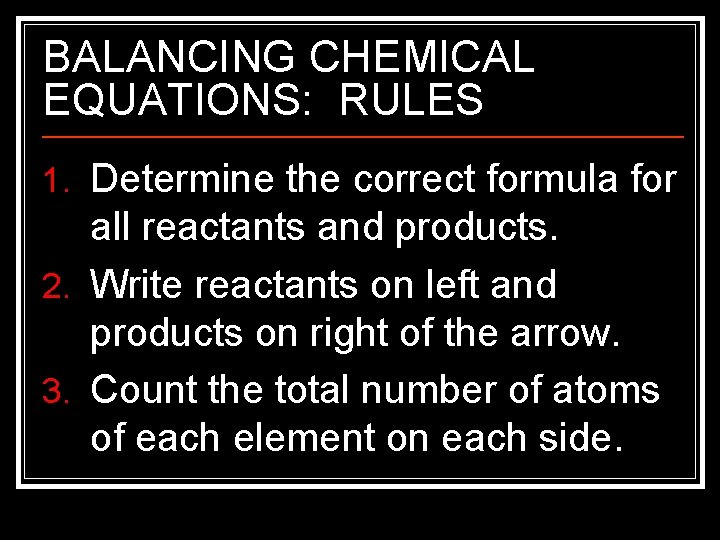BALANCING CHEMICAL EQUATIONS: RULES 1. Determine the correct formula for all reactants and products. 2. Write reactants on left and products on right of the arrow. 3. Count the total number of atoms of each element on each side.BALANCING CHEMICAL EQUATIONS: RULES 4. Balance the elements one at a time using coefficients – DO NOT CHANGE SUBSCRIPTS 5. Check each atom or polyatomic ion to make sure the equation is balanced.BALANCING CHEMICAL EQUATIONS: RULES 6. Make sure all coefficients are in the lowest possible ratio.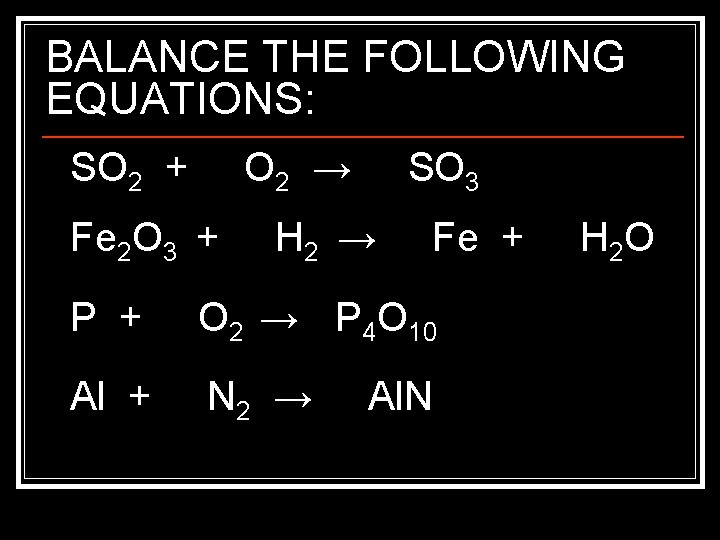BALANCE THE FOLLOWING EQUATIONS: SO 2 + O 2 → Fe 2 O 3 + SO 3 H 2 → Fe + P + O 2 → P 4 O 10 Al + N 2 → Al. N H 2 O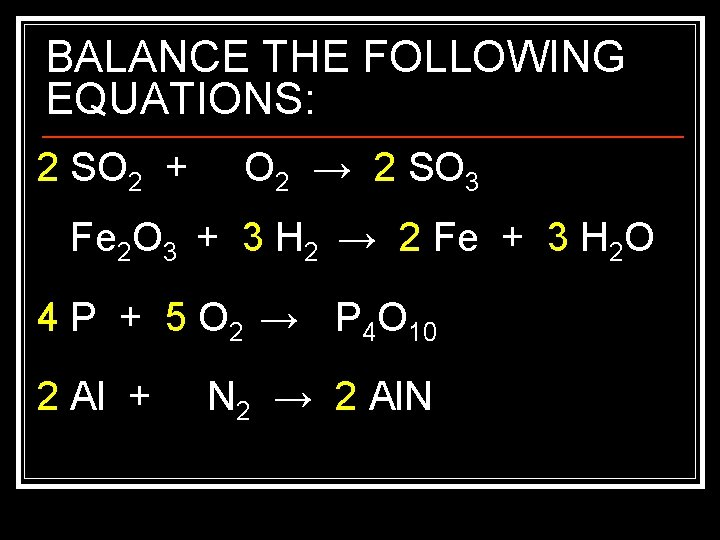BALANCE THE FOLLOWING EQUATIONS: 2 SO 2 + O 2 → 2 SO 3 Fe 2 O 3 + 3 H 2 → 2 Fe + 3 H 2 O 4 P + 5 O 2 → P 4 O 10 2 Al + N 2 → 2 Al. N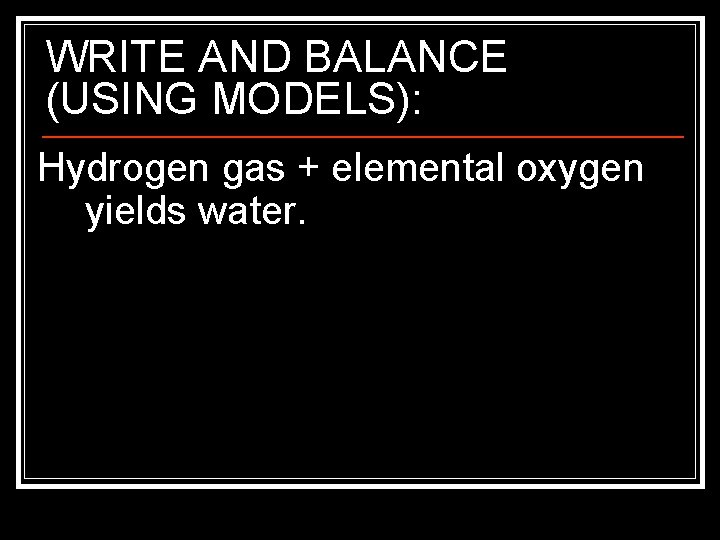WRITE AND BALANCE (USING MODELS): Hydrogen gas + elemental oxygen yields water.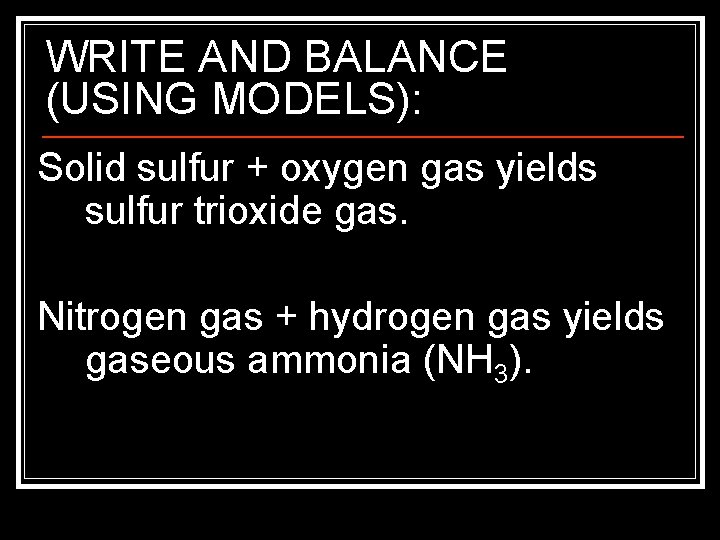WRITE AND BALANCE (USING MODELS): Solid sulfur + oxygen gas yields sulfur trioxide gas. Nitrogen gas + hydrogen gas yields gaseous ammonia (NH 3).